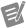# Solve Linear Equations (G Dataflow)

Last Modified: March 31, 2017

Solves a linear system Ax = y.## matrix type

Type of the input matrix.

Specifying the matrix type allows this node to execute more quickly by avoiding unnecessary computations, which could introduce numerical inaccuracy.

Name Description
General

The input matrix is a matrix that you cannot describe with one of the other categories.

Positive definite

The input matrix is positive-definite.

Lower triangular

The input matrix is lower triangular.

Upper triangular

The input matrix is upper triangular.

Default: General## matrix

A square or rectangular matrix.

This input accepts a 2D array of double-precision, floating point numbers or 2D array of complex double-precision, floating point numbers.

The number of rows in the real matrix must equal the number of elements in the known, dependent-variable values. Otherwise, the node returns an empty array for the solution vector and returns an error.

If the real matrix is singular and the matrix type is General, this node finds the least-square solution. Otherwise, this node returns an error when the real matrix is singular.## known vector

An array of known, dependent-variable values.

This input accepts the following data types:

• 1D array of double-precision, floating point numbers
• 1D array of complex double-precision, floating point numbers

The number of rows in the real matrix must equal the number of elements in the known, dependent-variable values. Otherwise, the node returns an empty array for the solution vector and returns an error.## error in

Error conditions that occur before this node runs.

The node responds to this input according to standard error behavior.

Standard Error Behavior

Many nodes provide an error in input and an error out output so that the node can respond to and communicate errors that occur while code is running. The value of error in specifies whether an error occurred before the node runs. Most nodes respond to values of error in in a standard, predictable way.

error in does not contain an error error in contains an errorIf no error occurred before the node runs, the node begins execution normally.

If no error occurs while the node runs, it returns no error. If an error does occur while the node runs, it returns that error information as error out.

If an error occurred before the node runs, the node does not execute. Instead, it returns the error in value as error out.

Default: No error## solution vector

The solution x to Ax = y where A is the input matrix and y is the known vector.

This output can return a matrix or a vector.## error out

Error information.

The node produces this output according to standard error behavior.

Standard Error Behavior

Many nodes provide an error in input and an error out output so that the node can respond to and communicate errors that occur while code is running. The value of error in specifies whether an error occurred before the node runs. Most nodes respond to values of error in in a standard, predictable way.

error in does not contain an error error in contains an errorIf no error occurred before the node runs, the node begins execution normally.

If no error occurs while the node runs, it returns no error. If an error does occur while the node runs, it returns that error information as error out.

If an error occurred before the node runs, the node does not execute. Instead, it returns the error in value as error out.

## Algorithm for Solving Linear Equations

Let A be an m-by-n matrix that represents matrix, y be the set of m coefficients that represents known vector, and x be the set of n elements that represents solution vector and solves the following system.

Ax = y

When m > n, the system has more equations than unknowns, which means the system is an over-determined system. Because the solution that satisfies Ax = y might not exist, this node finds the least-square solution x, which minimizes ||Ax - y||.

When m < n, the system has more unknowns than equations, which means the system is an under-determined system. The system may have infinite solutions that satisfy Ax = y. This node finds one of these solutions.

When m = n, if A is a nonsingular matrix (no row or column is a linear combination of any other row or column, respectively), then you can solve the system for x by decomposing A into its lower and upper triangular matrices, L and U, such that the following is true.

Ax = Lz = y

And z = Ux can be an alternate representation of the original system. Notice that z is also an n-element vector.

Triangular systems are easy to solve using recursive techniques. Consequently, when you obtain the L and U matrices from A, you can find z from the Lz = y system and x from the Ux = z system.

When mn, A can be decomposed to an orthogonal matrix Q and an upper triangular matrix R, so that A = QR. Then the linear system can be represented by QRx = y and you can solve Rx = QTy.

You can solve this triangular system to get x using recursive techniques.Note

You cannot always determine beforehand whether the matrix is singular, especially with large systems. This node detects singular matrices and returns an error if a singular matrix is found. You do not need to verify whether you have a valid system before using this node.

The numerical implementation of the matrix inversion is numerically intensive and, because of its recursive nature, is also highly sensitive to round-off error introduced by the floating-point numeric coprocessor. Although the computations use the maximum possible accuracy, this node cannot always solve the system.

Where This Node Can Run:

Desktop OS: Windows

FPGA: This product does not support FPGA devices